##### Statistics: 1,001 Practice Problems For DummiesA margin of error is the "plus or minus" part you have to add to your statistical results to tell everyone you acknowledge that sample results will vary from sample to sample, and could vary from the actual population condition. The margin of error helps you indicate how much you believe those results could vary, with a certain level of confidence. Solve the following problems about margin of error basics.

The following table provides the z*- values for selected (percentage) confidence levels.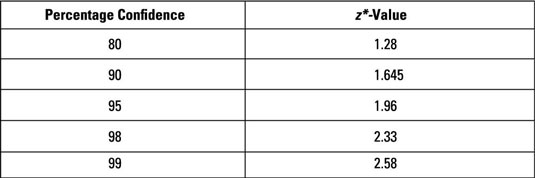## Sample questions

1. A poll shows that Garcia is leading Smith by 54% to 46% with a margin of error of plus/minus 5% at a 95% confidence level.

What conclusion can you draw from this poll?

Answer: The election is too close to call.

You can use the poll to conclude that 54% of the voters in this sample would vote for Garcia, and when you project the results to the population, you add a margin of error of plus/minus 5%. That means that the proportion voting for Garcia is estimated to be between 54% – 5% = 49% and 54% + 5% = 59% in the population with 95% confidence.

You can also use the poll to conclude that 46% of the voters in this sample would vote for Smith, and when you project the results to the population, you add a margin of error of plus/minus 5%. That means that the proportion voting for Smith is estimated to be between 46% – 5% = 41% and 46% + 5% = 51% in the population with 95% confidence (over many samples).

Garcia's confidence interval is 49% to 59%, and Smith's confidence interval is 41% to 51%. Because the confidence intervals overlap, the election is too close to call.

2. What is the margin of error for estimating a population mean given the following information and a confidence level of 95%?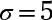n = 500

The formula for margin of error when estimating a population mean is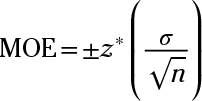where z* is the value from the table for a given confidence level (95% in this case, or 1.96),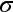is the population standard deviation (5), and n is the sample size (500).

Now, substitute the values into the formula and solve: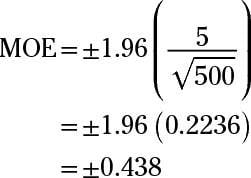The margin of error for a 95% confidence interval for the population mean is plus/minus 0.438.

If you need more practice on this and other topics from your statistics course, visit 1,001 Statistics Practice Problems For Dummies to purchase online access to 1,001 statistics practice problems! We can help you track your performance, see where you need to study, and create customized problem sets to master your stats skills.